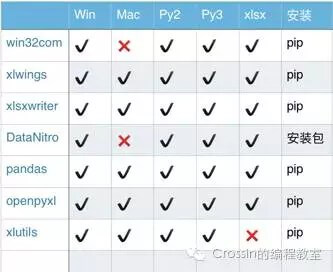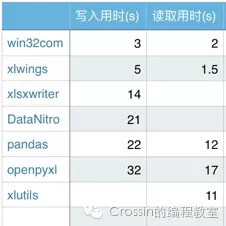目录

Python、Excel常用于数据处理，难免会产生相互的数据传递、计算处理。本文主要介绍Python-Excel系列的库，以及xlrd和xlwt两个库是使用。

## 1. 常用库

xlwings，openpyxl，pandas，win32com，xlsxwriter，DataNitro，xlutils

## 2. 环境要求• xlutils 仅支持 xls 文件，即2003以下版本
• win32com 与 DataNitro 仅支持 windows 系统
• xlwings 安装成功后，如果运行提示报错“ImportError: no module named win32api”，请再安装 pypiwin32 或者 pywin32 包
• win32com 不是独立的扩展库，而是集成在其他库中，安装 pypiwin32 或者 pywin32 包即可使用
• DataNitro 是 Excel 的插件，安装需到官网下载

## 3. 文档读写修改能力• xlsxwriter 不支持打开或修改现有文件
• xlwings 不支持对新建文件的命名
• DataNitro 作为 Excel 插件需依托于软件本身
• pandas 新建文档需要依赖其他库等等

## 4. 基本功能

• xlwings 可结合 VBA 实现对 Excel 编程，强大的数据输入分析能力，同时拥有丰富的接口，结合 pandas/numpy/matplotlib 轻松应对 Excel 数据处理工作。
• openpyxl 简单易用，功能广泛，单元格格式/图片/表格/公式/筛选/批注/文件保护等等功能应有尽有，图表功能是其一大亮点，缺点是对 VBA 支持的不够好。
• pandas 数据处理是 pandas 的立身之本，Excel 作为 pandas 输入/输出数据的容器。
• win32com 从命名上就可以看出，这是一个处理 windows 应用的扩展，Excel 只是该库能实现的一小部分功能。该库还支持 office 的众多操作。需要注意的是，该库不单独存在，可通过安装 pypiwin32 或者 pywin32 获取。
• xlsxwriter 拥有丰富的特性，支持图片/表格/图表/筛选/格式/公式等，功能与openpyxl相似，优点是相比 openpyxl 还支持 VBA 文件导入，迷你图等功能，缺点是不能打开/修改已有文件，意味着使用 xlsxwriter 需要从零开始。
• DataNitro 作为插件内嵌到 Excel 中，可完全替代 VBA，在 Excel 中使用 python 脚本。既然被称为 Excel 中的 python，协同其他 python 库亦是小事一桩。然而，这是付费插件…
• xlutils 基于 xlrd/xlwt，老牌 python 包，算是该领域的先驱，功能特点中规中矩，比较大的缺点是仅支持 xls 文件

## 5. 性能

分别使用不同库进行添加及读取 1000行 * 700列 数据操作，得到所用时间，重复操作取平均值## 6. 库选择建议

• 不想使用 GUI 而又希望赋予 Excel 更多的功能，openpyxl 与 xlsxwriter，你可二者选其一
• 需要进行科学计算，处理大量数据，建议 pandas+xlsxwriter 或者 pandas+openpyxl；
• 想要写 Excel 脚本，会 Python 但不会 VBA ，可考虑 xlwings 或 DataNitro；

## 7. 使用xlrd、xlwt读写excel

# coding=utf-8
def list_wirte_to_excel(data_list):
'''
:param data_list = [(1, 2, 3),(11, 21, 31)]:
'''
import xlwt
excel = xlwt.Workbook(encoding='utf-8')
sheet1 = excel.add_sheet(u'sheet1', cell_overwrite_ok=True)  # 创建sheet1
columns = [u'第一列', u'第二列', u'时间']

# 创建列名栏
for i in xrange(0, len(columns)):
sheet1.write(0, i, columns[i])

# 写入数据
for i in xrange(0, len(data_list)):
if len(data_list[i]) == len(columns):
# write(行，列，数据，样式)
sheet1.write(i + 1, 0, data_list[i])
sheet1.write(i + 1, 1, data_list[i])
sheet1.write(i + 1, 2, data_list[i])
excel.save('excel.xls')

def excel_to_list(excel_path):
'''
:param excel_path 能访问的excel路径:
:return包含全部数据的list:[(第一列数据), (第二列数据)]
'''
import xlrd
wb = xlrd.open_workbook(excel_path)
# 两种方式：索引和名字
sheet = wb.sheet_by_index(0)

data = [sheet.row_values(rownum) for rownum in xrange(sheet.nrows)]

# 如果只想返回第一列数据：
# sheet.col_values(0)
# 通过索引读取数据
# cell(行,列), 获取第一行，第一列数据
# sheet.cell(0, 0).value
return data[1:]

if __name__ == '__main__':
import random
import datetime
data = [(random.randint(0, 1000), random.randint(0, 1000), datetime.datetime.now().strftime('%Y-%m'))
for i in xrange(1000)]
list_wirte_to_excel(data)

print excel_to_list('./excel.xls')